## Helmholtz Differential Equation Polar Coordinates

In 2-D Polar Coordinates, attempt Separation of Variables by writing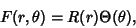(1)

then the Helmholtz Differential Equation becomes(2)

Divide both sides by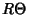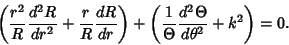(3)

The solution to the second part of (3) must be periodic, so the differential equation is(4)

which has solutions(5)

Plug (4) back into (3)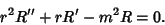(6)

This is an Euler Differential Equation withand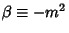. The roots are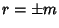. So for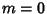,and the solution is(7)

But since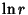blows up at, the only possible physical solution is. When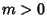,, so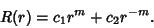(8)

But sinceblows up at, the only possible physical solution is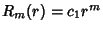. The solution foris then(9)

for, 1, ...and the general solution is(10)

References

Morse, P. M. and Feshbach, H. Methods of Theoretical Physics, Part I. New York: McGraw-Hill, pp. 502-504, 1953.